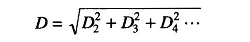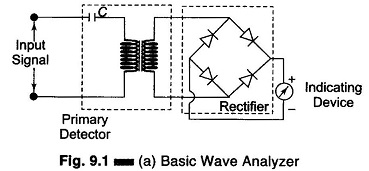## What is Wave Analyzer?:

It can be shown mathematically that any complex waveform is made up of a fundamental and its harmonics. It is often desired to measure the amplitude of each harmonic or fundamental individually. This can be performed by instruments called wave analyzers. This is the simplest form of analysis in the frequency domain, and can be performed with a set of tuned filters and a voltmeter. Wave analyzers are also referred to as frequency selective voltmeters, carrier frequency voltmeters, and selective level voltmeters. The Basic wave analyzer instrument is tuned to the frequency of one component whose amplitude is measured.

This instrument is a narrow band superheterodyne receiver, similar to a spectrum analyzer (discussed later). It has a very narrow pass-band. A meter is used for measurement, instead of a CRT. Wave analyzers are used in the low RF range, below 50 MHz and down through the AF range. They provide a very high frequency resolution.

Some wave analyzers have the facility of automatic frequency control, in which the tuning automatically locks to a signal. This makes it possible to measure the amplitude of signals that are drifting in frequency by amounts that would carry them outside the widest pass-band available.

When a sinusoidal signal is applied to the input of an ideal linear amplifier, it produces a sinusoidal output waveform. However, in most cases the output waveform is not an exact replica of the input signal because of different types of distortion. The amount by which the output waveform of an amplifier differs from the input waveform is a measure of the distortion introduced by the inherent non-linear characteristics of the active devices.

Harmonic distortion analyzers measure the total harmonic content in the waveforms. It can be shown mathematically that an amplitude distorted sine wave is made up of pure sine wave components, including the fundamental frequency f of the input signal, and harmonic multiples of the fundamental fre­quency, 2f, 3f, 4f etc.

Harmonic distortion can be quantitatively measured very accurately with a harmonic distortion analyzer, generally called a distortion analyzer.

The total harmonic distortion or factor is given bywhere D2, D2, D3 … represent the second harmonic, third harmonic, etc. re­spectively.

The distortion analyzer measures the total harmonic distortion without indi­cating the amplitude and frequency of each component waves.

Signal analysis of both random and periodic signals in the frequency domain is used extensively in electronic and telecommunications. The frequency stabil­ity and spectral purity of signal sources can be measured by the use of these signal analyzers.

These signal analyzers can be used along with a frequency generator or a source of white or pseudo-random noise to measure the frequency response of amplifiers, filters or other networks.

The operational characteristics of a transreceiver and communication system are determined by measuring various parameters, such as spectral purity of the carrier wave, spectral power distribution of the amplitude or frequency modu­lated wave, signal distortion, and the systems signal to noise ratio.

Such analysis is provided by a wave analyzer, distortion analyzer, spectrum analyzer, and digital fourier analyzer.

### Block Diagram of Basic Wave Analyzer:

A basic wave analyzer is shown in Fig. 9.1(a). It consists of a primary detector, which is a simple LC circuit. This LC circuit is adjusted for resonance at the frequency of the particular harmonic component to be measured.The intermediate stage is a full wave rectifier, to obtain the average value of the input signal. The indicating device is a simple dc voltmeter that is calibrated to read the peak value of the sinusoidal input voltage.

Since the LC circuit is tuned to a single frequency, it passes only the frequency to which it is tuned and rejects all other frequencies. A number of tuned filters, connected to the indicating device through a selector switch, would be required for a useful Wave analyzer.

### Types of Wave Analyzer:

There are two types of Wave Analyzer namely,

1. Frequency Selective Wave Analyzer
2. Heterodyne Wave Analyzer

### Applications of Wave Analyzer:

• It is used to measures the harmonic distortion of the signal
• It is used to measuring the amplitude of the selected frequency component in the signal
• It is used to decrease the sound and Vibration from electrical machines
• It is used as a harmonic distortion analyzer
• It is used for analyzing the DC Component with periodic signals
• It is used as a automatic frequency controller
• It is used in electrical measurements
Scroll to Top